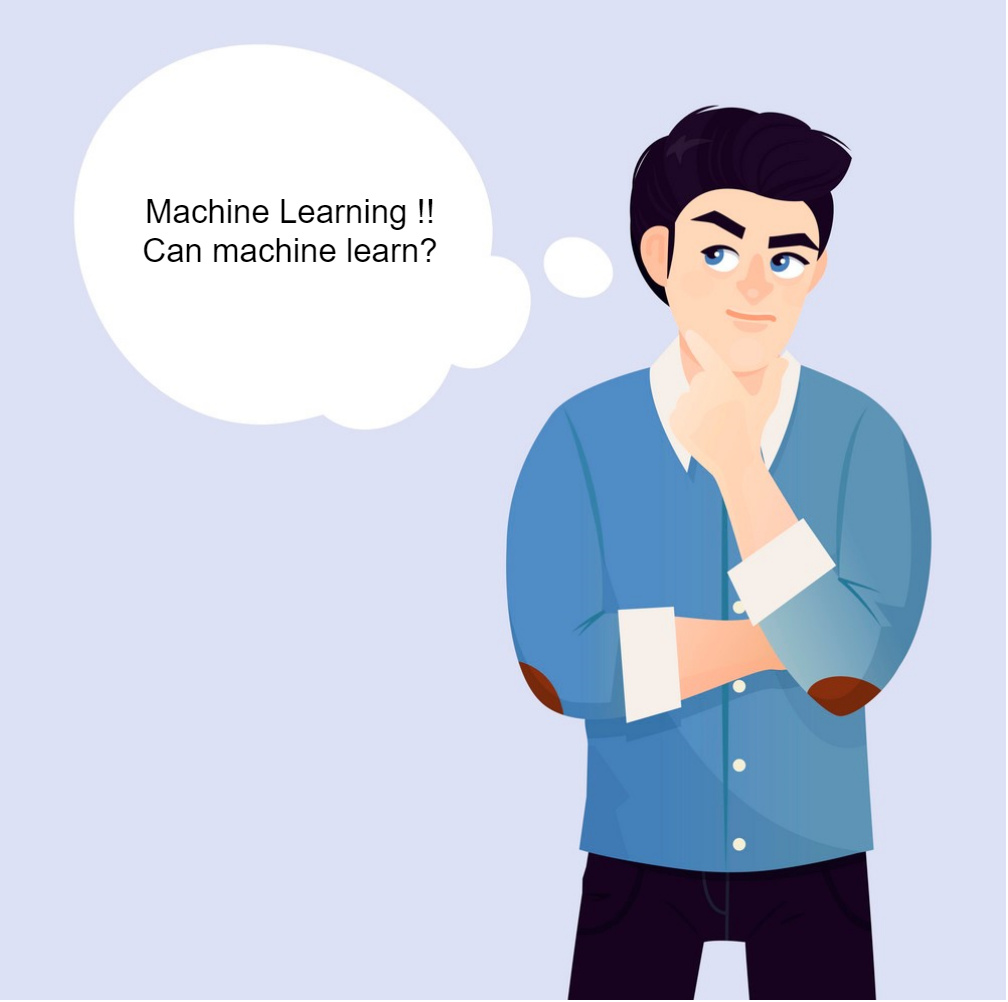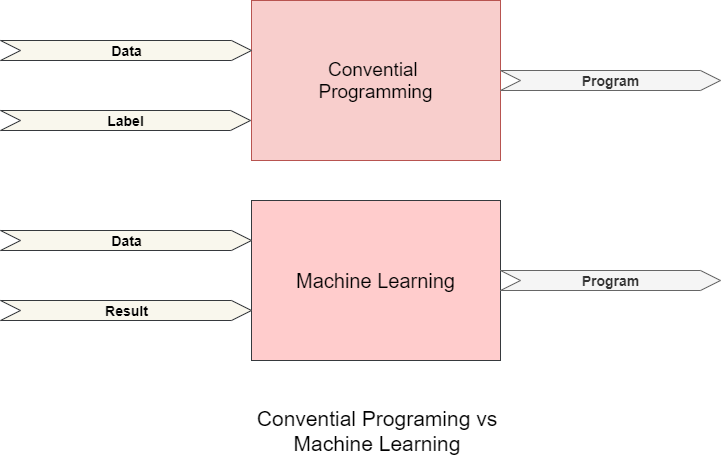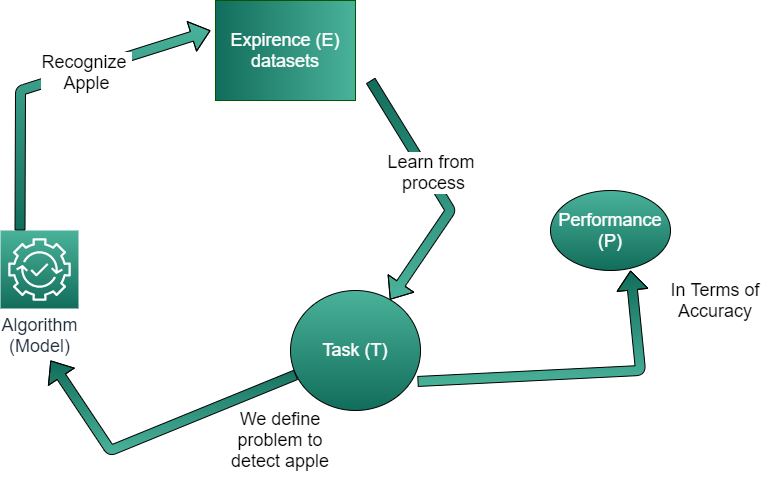# Introduction of machine learningI will begin the machine learning series with this blog. First we will walk through basic concepts for building the foundation and we will walk with implementation code. We can found lots of content defining the machine learning which are complicated to grasp for the beginners. I will try to elaborate in more understandable and simplified form.

# Defination:

let's start with the simple concept of human learning process. let's observe the following picture and say what it is.You can say apple, right? Sounds silly question and it may be. But do you know how you are able to recognise this as apple? It 's simply because you have been taught to pronuce this as an apple. In other words you are taught to recognise this fruit as an apple.

Now let's go on more educational appraoch, here picture represents the data, apple is the result and your thought process acts as algorithm.Now we can summarize your learning process here, you observe the objects, you have the result (name of object)  and you use your brain to process, memorize and recognize this object as an apple. We simply try to implement this learning technique for machine to learn.

Now we will go through difference between convential programing and machine learning. Figure illustrate alot here. Though i will try to illustrate few highlights.### Conventional programing:

We have lots of data,then we have mathmatical models to process the data and we observe the results based upon this mathemetical model.

### Machine learning:

in this case, we use data with results and let the machine define/derive the algorithm so as to develop the model for future prediction based upon this expirence.

Now let's dive in to the actual defination of machine learning. We will define the problem to recognise apple. We try to understand the concept with following diagram:We try to make machine (algorithm) learn from the Expirence(E) for correctly detecting an apple where problem (task T) is measured by performance (P) in terms of accuracy of correctly detecting an Apple.

Now you have understood the concept of machine learning we will move forward to next step with next post.